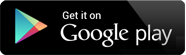## Video Lectures

Chapter 1 : Functions
Topic : Definition of functions, Domain, co-domain and range of function, Direct and inverse images
Content : Injective, surjective and bijective functions, Composite and inverse functions 73 MB ,53:59 MIN , THEORY

Chapter 1 : Functions
Topic : Examples of standard functions
Content : Identity Function, Constant Function, Absolute Function, Logarithmic and Exponential Functions, Flooring and ceiling functions 35 MB ,27:34 MIN , THEORY

Chapter 2 : Relations
Topic : Definition and examples of relation, Representation of Relation using diagraphs and matrices
Content : Representation of Relation using diagraphs and matrices 76 MB ,53:56 MIN , THEORY

Chapter 2 : Relations
Topic : Properties of Relations, Equivalence relation, Partial ordering relation, Hasse diagram
Content : Examples of Equivalence Relation and partial order Relation 82 MB ,58:34 MIN , THEORY

Chapter 2 : Relations
Topic : Maximal, minimal, greatest, least element, Lattices
Content : Dual lattice, distributive lattice, modular lattice, complemented lattice 169 MB ,2:0:51 MIN , THEORY

Chapter 3 : Recurrence Relations
Topic : Definition of recurrence Relations, Formulating Recurrence relation, Solution of recurrence relation
Content : Degree of Recurrence relation, Characteristic Roots of Recurrence Relation 88 MB ,1:4:10 MIN , THEORY

Chapter 3 : Recurrence Relations
Topic : Solving recurrence relations-Back tracking method, Linear homogeneous recurrence relations with constant, Homogeneous solution of linear homogeneous recurrence relation with constant coefficients, particular solution of non-linear homogeneous recurrence relation with constant coefficients, General solution of non- linear homogeneous recurrence relation with constant coefficients, Applications- Formulate and solve recurrence relation for Fibonacci numbers, Tower of Hanoi, Intersection of lines in a plane
Content : Sorting Algorithms 76 MB ,53:49 MIN , THEORY

Chapter 1 : Counting Principles
Topic : Basic counting principles (sum and product rule); Pigeonhole principle (without proof)- simple examples; Inclusion Exclusion principle (Sieve formula) (without proof); Counting Tree Diagrams
Content : Inclusion Exclusion Principle for three sets 46 MB ,30:23 MIN , THEORY

Chapter 2 : Permutations and Combinations
Topic : Permutation without and with repetition; Combination without and with Repetition; Binomial number and Identities: Pascal Identity, Vandermonde's Identity, Pascal Triangle, Binomial theorem (without proof) and applications; Multinomial numbers, Multinomial Theorem( without proof) and applications
Content : Pascal Triangle 47 MB ,32:33 MIN , THEORY

Chapter 3 : Languages, Grammars and Machines
Topic : Languages and Grammars - Introduction, Phase structure grammar, Types of Grammar, Derivation trees, Finite-state machines with output; Finite-state machines with no output; Regular Expression and regular Language.
Content : Derivation trees 29 MB ,22:3 MIN , THEORY

Chapter 1 : Graphs
Topic : Graph and Graph models, Graph terminologies and Special types of graph, Definitions and Elementary results, Representing Graphs, Graph Isomorphisms, Connectivity in Graph-path, trail, walk, Euler and Hamiltonian paths, Planar graphs
Content : Hand Shaking lemma 87 MB ,59:8 MIN , THEORY

Chapter 1 : Graphs
Topic : Linked representation of a graph, Graph coloring and Chromatic number
Content : Chromatic number of cycle, planar graph, complete graph 23 MB ,16:33 MIN , THEORY

Chapter 2 : Trees
Topic : Definitions, Tree terminology and elementary results, linked representation of binary trees, ordered rooted tree, Binary trees, Complete and extended binary tree, Expression trees, Binary search tree, Algorithm for searching and inserting in binary search trees, Algorithms for deleting in a binary search tree
Content : Level of root, Height of tree 29 MB ,19:57 MIN , THEORY

Chapter 2 : Trees
Topic : Traversing binary trees
Content : Pre-order traversal, In-order traversal, Post-order traversal 13 MB ,10:10 MIN , THEORY

## The Shikshak App

The Shikshak App is an honest attempt to provide quality education.